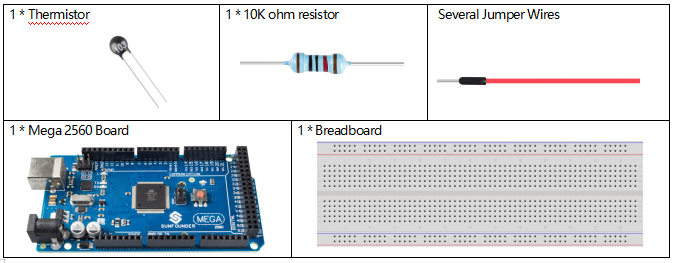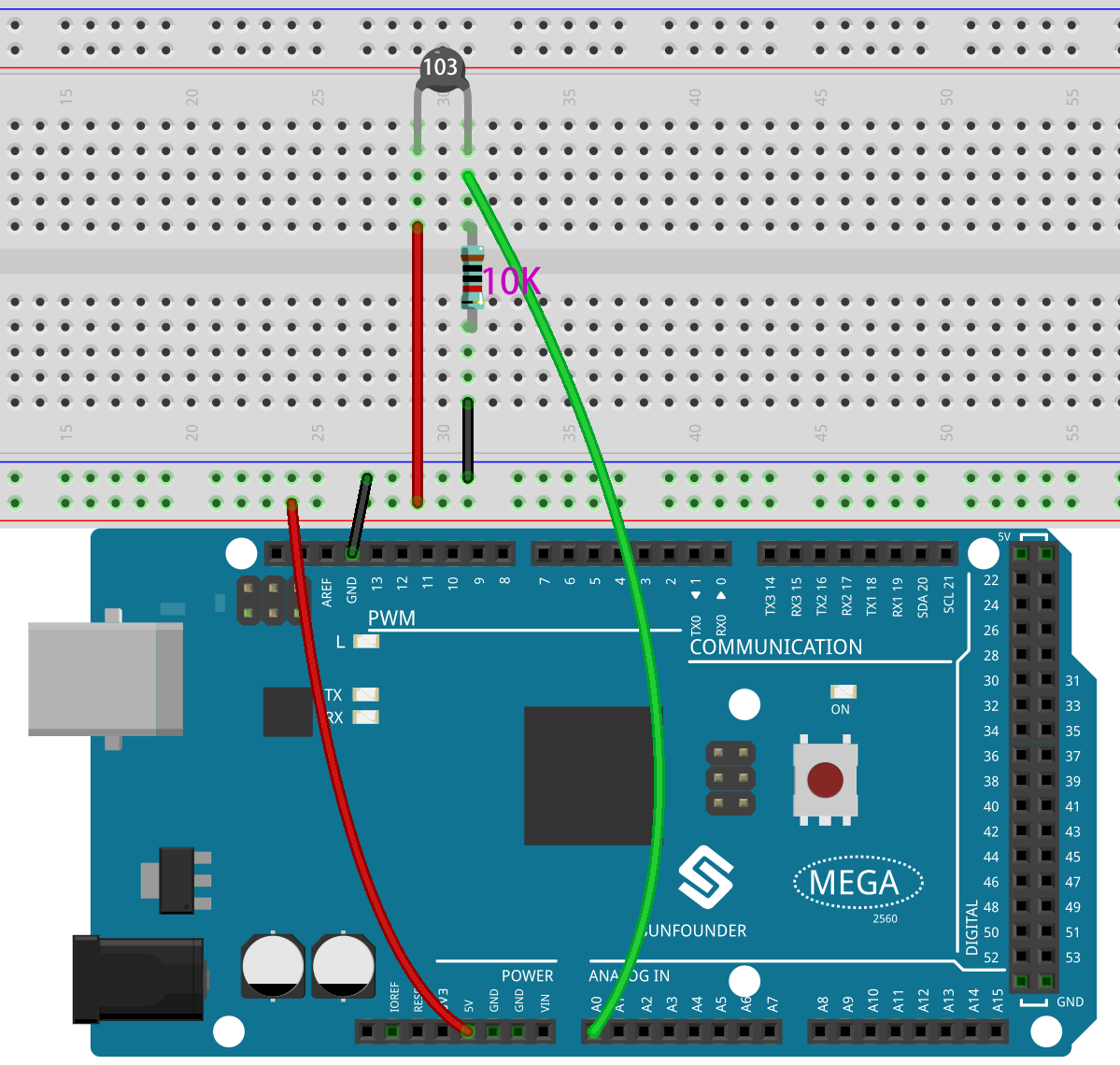2.27 Thermistor¶

Overview¶

In this lesson, you will learn how to use thermistor. Thermistor can be used as electronic circuit components for temperature compensation of instrument circuits. In the current meter, flowmeter, gas analyzer, and other devices. It can also be used for overheating protection, contactless relay, constant temperature, automatic gain control, motor start, time delay, color TV automatic degaussing, fire alarm and temperature compensation.

Components Required¶Component Introduction¶Thermistor is a sensitive element, and it has two types: Negative Temperature Coefficient (NTC) and Positive Temperature Coefficient (PTC), also known as NTC and PTC. Its resistance varies significantly with temperature. The resistance of PTC thermistor increases with temperature ,while the condition of NTC is opposite to the former. In this experiment we use NTC.

The principle is that the resistance of the NTC thermistor changes with the temperature of the outer environment. It detects the real-time temperature of the environment. When the temperature gets higher, the resistance of the thermistor decreases. Then the voltage data is converted to digital quantities by the A/D adapter. The temperature in Celsius or Fahrenheit is output via programming.

In this experiment, a thermistor and a 10k pull-up resistor are used. Each thermistor has a normal resistance. Here it is 10k ohm, which is measured under 25 degree Celsius.

Here is the relation between the resistance and temperature:

RT =RN expB(1/TK – 1/TN)

RT is the resistance of the NTC thermistor when the temperature is TK.

RN is the resistance of the NTC thermistor under the rated temperature TN. Here, the numerical value of RN is 10k.

TK is a Kelvin temperature and the unit is K. Here, the numerical value of TKis 273.15 + degree Celsius.

TN is a rated Kelvin temperature; the unit is K too. Here, the numerical value of TN is 273.15+25.

And B(beta), the material constant of NTC thermistor, is also called heat sensitivity index with a numerical value 3950.

exp is the abbreviation of exponential, and the base number e is a natural number and equals 2.7 approximately.

Convert this formula TK=1/(ln(RT/RN)/B+1/TN) to get Kelvin temperature that minus 273.15 equals degree Celsius.

This relation is an empirical formula. It is accurate only when the temperature and resistance are within the effective range.

Fritzing Circuit¶

In this example, we use the analog pin A0 to get the value of Thermistor. One pin of thermistor is connected to 5V, and the other is wired up to A0. At the same time, a 10kΩ resistor is connected with the other pin before connecting to GND.Code¶

After uploading the code to the Mega2560 board, you can open the serial monitor to check the current temperature. The Kelvin temperature is calculated according to the formula TK=1/(ln(RT/RN)/B+1/TN).